Test: AC Voltage Controllers

# Test: AC Voltage Controllers

Test Description

## 30 Questions MCQ Test GATE Electrical Engineering (EE) 2023 Mock Test Series | Test: AC Voltage Controllers

Test: AC Voltage Controllers for Electrical Engineering (EE) 2023 is part of GATE Electrical Engineering (EE) 2023 Mock Test Series preparation. The Test: AC Voltage Controllers questions and answers have been prepared according to the Electrical Engineering (EE) exam syllabus.The Test: AC Voltage Controllers MCQs are made for Electrical Engineering (EE) 2023 Exam. Find important definitions, questions, notes, meanings, examples, exercises, MCQs and online tests for Test: AC Voltage Controllers below.
Solutions of Test: AC Voltage Controllers questions in English are available as part of our GATE Electrical Engineering (EE) 2023 Mock Test Series for Electrical Engineering (EE) & Test: AC Voltage Controllers solutions in Hindi for GATE Electrical Engineering (EE) 2023 Mock Test Series course. Download more important topics, notes, lectures and mock test series for Electrical Engineering (EE) Exam by signing up for free. Attempt Test: AC Voltage Controllers | 30 questions in 25 minutes | Mock test for Electrical Engineering (EE) preparation | Free important questions MCQ to study GATE Electrical Engineering (EE) 2023 Mock Test Series for Electrical Engineering (EE) Exam | Download free PDF with solutions
 1 Crore+ students have signed up on EduRev. Have you?
Test: AC Voltage Controllers - Question 1

### AC voltage controllers convert

Detailed Solution for Test: AC Voltage Controllers - Question 1

Voltage controllers convert the fixed ac voltage to variable ac by changing the values of the firing angle.

Test: AC Voltage Controllers - Question 2

### In AC voltage controllers the

Detailed Solution for Test: AC Voltage Controllers - Question 2

Voltage controllers convert the fixed ac voltage to variable ac by changing the values of the firing angle. The available ac obtained has the same fixed frequency as the input ac.

Test: AC Voltage Controllers - Question 3

### Earlier then the semiconductor technology, ___________ devices were used for voltage control applications.

Detailed Solution for Test: AC Voltage Controllers - Question 3

A tap changing transformer can give variable ac from fixed ac without a change in frequency.

Test: AC Voltage Controllers - Question 4

The AC voltage controllers are used in __________ applications.

Detailed Solution for Test: AC Voltage Controllers - Question 4

In electric heating, variable ac supply is needed. The devices are fired appropriately to apply enough temperature.

Test: AC Voltage Controllers - Question 5

In the principle of phase control

Detailed Solution for Test: AC Voltage Controllers - Question 5

Switching devices is so operated that the load gets connected to ac source for a part of each half cycle.

Test: AC Voltage Controllers - Question 6

A single-phase half wave voltage controller consists of

Detailed Solution for Test: AC Voltage Controllers - Question 6

As it is half wave, it consists of one SCR ( the control element) in anti parallel with one diode.

Test: AC Voltage Controllers - Question 7

In the below given voltage controller circuit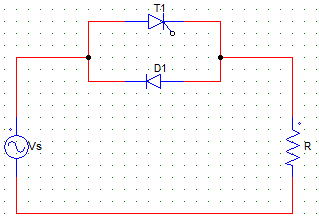Detailed Solution for Test: AC Voltage Controllers - Question 7

As T1 is triggered at an angle α, the conduction in the positive have cycle will start at α. In the negative half cycle, the diode is forward biased and load is connected as it is to the supply.

Test: AC Voltage Controllers - Question 8

The below shown controller circuit is a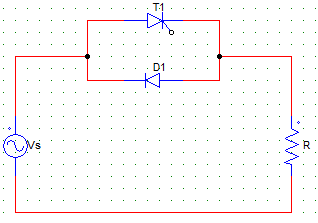Detailed Solution for Test: AC Voltage Controllers - Question 8

As it consists one diode and one SCR only, the control is only in one cycle (the positive half in this case), hence it is a half wave controller.

Test: AC Voltage Controllers - Question 9

The below given controller circuit is a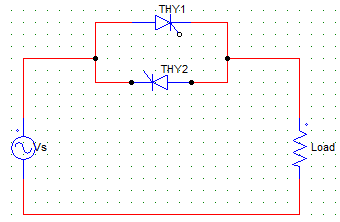Detailed Solution for Test: AC Voltage Controllers - Question 9

As it consists of two SCRs, it is a full wave controller.

Test: AC Voltage Controllers - Question 10

In the below given voltage controller circuit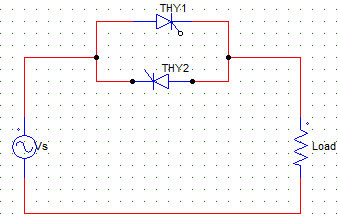Detailed Solution for Test: AC Voltage Controllers - Question 10

As it consists of two SCRs, it is a full wave controller and both the half cycles can be controlled by varying their respective firing angles.

Test: AC Voltage Controllers - Question 11

In the below shown circuit, the diode conducts for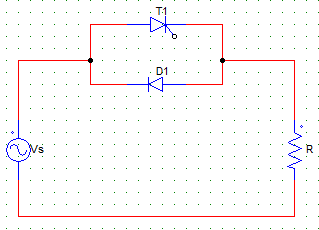Detailed Solution for Test: AC Voltage Controllers - Question 11

The diode conducts from π to 2π in the negative half cycle. If it were a non resistive load, the diode would conduct for less than 90°, as the inductor would force conduct the SCR for some time.

Test: AC Voltage Controllers - Question 12

The SCR T1 is fired at an angle of α, and the supply Vs = Vm sinωt. Find the average value of the output voltage.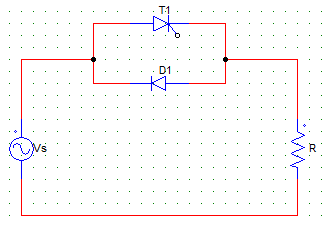Detailed Solution for Test: AC Voltage Controllers - Question 12

Vo = (1/2π) ∫Vm sinωt d(ωt). Where the integration would be run from α to 2π as the conduction takes place from α to 2π.
Vo = (Vm/2π) (cosα – 1).

Test: AC Voltage Controllers - Question 13

The below given output voltage waveform can be obtained by a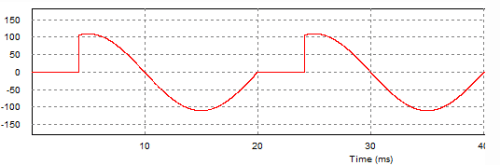Detailed Solution for Test: AC Voltage Controllers - Question 13

As the positive half is chopped off due to some value of firing angle, the firing angle is not equal to zero. As the negative half is a half sine wave, either it is a full wave controller with firing angle for T2 set to zero or it is a half wave controller with a Thyristor (T1) and a diode.

Test: AC Voltage Controllers - Question 14

In the below shown AC converter circuit with firing angle = α for both the devices, T2 will conduct from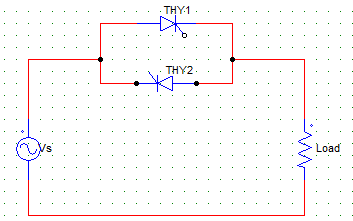Detailed Solution for Test: AC Voltage Controllers - Question 14

T2 is triggered at π + α, it conducts from π + α to 2π after which it is line commutated.

Test: AC Voltage Controllers - Question 15

The below given output voltage waveform can be obtained by a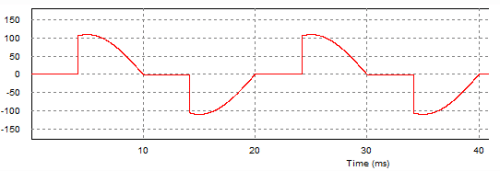Detailed Solution for Test: AC Voltage Controllers - Question 15

As the control is in both the directions or cycles, it is a full wave ac voltage controller circuit.

Test: AC Voltage Controllers - Question 16

In the integral cycle control method of ac voltage controller

Detailed Solution for Test: AC Voltage Controllers - Question 16

In integral cycle control, power is delivered for m cycles and not delivered for n cycles. Hence, the average value of the power delivered is controlled by manipulating m and n.

Test: AC Voltage Controllers - Question 17

In the integral cycle control of ac voltage controller, is the load is on for n cycles and off for m cycles, then the periodicity is given by? Consider the output is sinusoidal.

Detailed Solution for Test: AC Voltage Controllers - Question 17

Over a complete cycle of 2π x (on cycles + off cycles) the power is delivered for n cycles.
Hence, P = n/2π(m+n).

Test: AC Voltage Controllers - Question 18

If k is the duty cycles of the controller, then the rms value of the output voltage in case of a integral cycle control circuit will be?
Consider the input to be sinusoidal with peak value Vm and rms value Vs.

Detailed Solution for Test: AC Voltage Controllers - Question 18

Vrms = [ (k/2π) x ∫Vmsin⁡2 ωt d(ωt) ] 1/2
Where the integral runs from 0 to 2π.
Vrms = (Vm/√2) k = Vs x √k.

Test: AC Voltage Controllers - Question 19

Find the power delivered to the load in the integral cycle control method of ac voltage control, having a sine input of Vs, R load and duty cycle = k.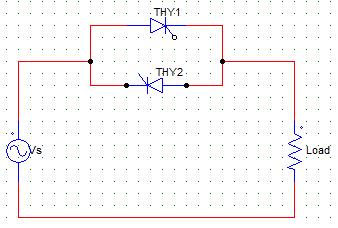Detailed Solution for Test: AC Voltage Controllers - Question 19

Output voltage = Vs x √k
P = (Vs x √k)2/R.

Test: AC Voltage Controllers - Question 20

In the integral cycle control method with duty cycle = k and maximum load current = Im. Find the value of average SCR current.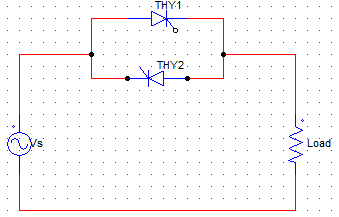Detailed Solution for Test: AC Voltage Controllers - Question 20

As each SCR conducts for π radians during each cycle of n on cycles, the average value of SCR current is
I = (k/2π) ∫Im sin ωt d(ωt). Where the integration is from 0 to π.

Test: AC Voltage Controllers - Question 21

The below given circuit has Vs = 230V and R = 20 Ω. Find the value of the average output voltage at the R load for a firing angle of 45°.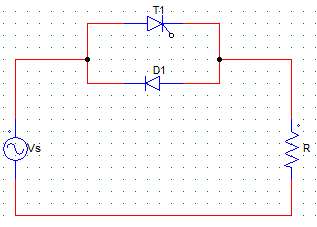Detailed Solution for Test: AC Voltage Controllers - Question 21

Vo = [(√2 x 230) x (cos45 – 1)]/2π = -15.17 V.
Negative value is due to the fact that the average value in the positive half cycle is less than that in the negative half cycle.

Test: AC Voltage Controllers - Question 22

The below given circuit has Vs = 230V and R = 20 Ω. Find the value of the average output load current at the R load for a firing angle of 45°.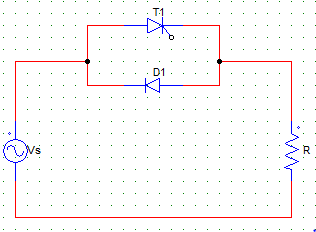Detailed Solution for Test: AC Voltage Controllers - Question 22

Vo = [(√2 x 230) x (cos45 – 1)]/2π = -15.17 V.
Io = (Vo)/R = -0.7585
Negative value is due to the fact that the average value in the positive half cycle is less than that in the negative half cycle.

Test: AC Voltage Controllers - Question 23

A single phase voltage controller has input of 230 V and a load of 15 Ω resistive. For 6 cycles on and 4 cycles off, determine the rms output voltage.

Detailed Solution for Test: AC Voltage Controllers - Question 23

Vrms = Vo x √k
k = (6/6+4) = 6/10 = 0.6
Vrms = √0.6 x 230 = 178.157 V.

Test: AC Voltage Controllers - Question 24

A single phase voltage controller has input of 230 V and a load of 15 Ω resistive. For 6 cycles on and 4 cycles off, determine the input pf.

Detailed Solution for Test: AC Voltage Controllers - Question 24

k = (6/6+4) = 6/10 = 0.6
input pf = √0.6 = 0.7746.

Test: AC Voltage Controllers - Question 25

A single phase voltage controller has input of 230 V and a load of 15 Ω resistive. For 6 cycles on and 4 cycles off, determine the power delivered to the load.

Detailed Solution for Test: AC Voltage Controllers - Question 25

Vrms = Vo x √k
k = (6/6+4) = 6/10 = 0.6
Vrms = √0.6 x 230 = 178.157 V
P = (Vrms)2/R = 2116 W = 2.116 kW.

Test: AC Voltage Controllers - Question 26

A single phase voltage controller has input of 230 V and a load of 15 Ω resistive. For 6 cycles on and 4 cycles off, determine the average value of SCR current.

Detailed Solution for Test: AC Voltage Controllers - Question 26

Peak current Im = (230 x √2)/15 = 21.681 A
k = (6/6+4) = 6/10 = 0.6
Avg current = (k Im)/π = 4.14 A.

Test: AC Voltage Controllers - Question 27

Pulse gating is suitable for

Detailed Solution for Test: AC Voltage Controllers - Question 27

In RL loads with pulse gating, the incoming SCR may be fired during the interval when it is reversed biased by the outgoing SCR, thus it won’t get turn on even after the outgoing SCR is not reveres basing the incoming SCR is the pulse width is over before forward biasing the SCR.

Test: AC Voltage Controllers - Question 28

In continues gating

Detailed Solution for Test: AC Voltage Controllers - Question 28

As the gating is applied for a longer duration, the device is heated up.

Test: AC Voltage Controllers - Question 29

High frequency gating uses a

Detailed Solution for Test: AC Voltage Controllers - Question 29

In high frequency gating a train of pulses are used to overcome the thermal problems due to continuous gating.

Test: AC Voltage Controllers - Question 30

A single-phase voltage controller, using one SCR in anti parallel with a diode, feeds a load R and Vs = 230 V. For a firing angel of 90° for the SCR, the PMMC voltage connected across R would read

Detailed Solution for Test: AC Voltage Controllers - Question 30

As firing angle is 90, there is ideally be no conduction in the positive half. Hence, the average value will be zero.
Vo = (√2 Vs)/2π x (cos90 – 1) = – 51.8 V.

## GATE Electrical Engineering (EE) 2023 Mock Test Series

21 docs|273 tests
 Use Code STAYHOME200 and get INR 200 additional OFF Use Coupon Code
Information about Test: AC Voltage Controllers Page
In this test you can find the Exam questions for Test: AC Voltage Controllers solved & explained in the simplest way possible. Besides giving Questions and answers for Test: AC Voltage Controllers, EduRev gives you an ample number of Online tests for practice

## GATE Electrical Engineering (EE) 2023 Mock Test Series

21 docs|273 tests